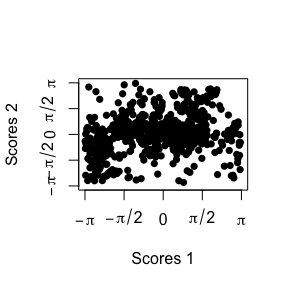# ridgetorus: PCA on the Torus via Density Ridges

#### 2023-01-09, v1.0.1

In a wide spectrum of applied fields, data is present as multivariate circular data (toroidal data), rendering spurious the application of many standard statistical tools. The package ridgetorus implements Toroidal Ridge PCA (TR-PCA), an alternative to PCA suited to toroidal data (García-Portugués and Prieto-Tirado 2022). Below are some simple examples of the use of TR-PCA through ridge_pca(), the main function in ridgetorus.

## Texan wind

The following example illustrates the application of TR-PCA to a small dataset comprised by wind directions measured at 6:00 and 7:00 from June 1, 2003 to June 30, 2003 at a weather station in Texas. The direction is measured in radians in $$[-\pi, \pi)$$ with $$-\pi/-\frac{\pi}{2}/0/\frac{\pi}{2}/\pi$$ representing the East/South/West/North/East directions.

library(ridgetorus)
data("wind")
plot(wind, xlim = c(-pi, pi), ylim = c(-pi, pi), axes = FALSE,
xlab = expression(theta), ylab = expression(theta))
sdetorus::torusAxis()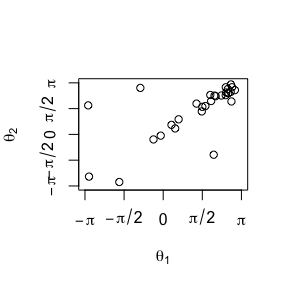TR-PCA works by first modeling the data with the best-fitting model among a Bivariate Wrapped Cauchy (Kato and Pewsey 2015) and a Bivariate Sine von Mises (Singh, Hnizdo, and Demchuk 2002). Then, the density ridge (see Genovese et al. (2014)) is computed and its connected component through the mode(s) of the distribution is extracted. In this case, this connected component explains $$62\%$$ of the variance of the original data. The plot below shows how the scores are computed, and how their signs are assigned. The blue asterisk stands for the Fréchet mean of the sample through TR-PCA.

# Fit TR-PCA
fit <- ridge_pca(x = wind)
show_ridge_pca(fit)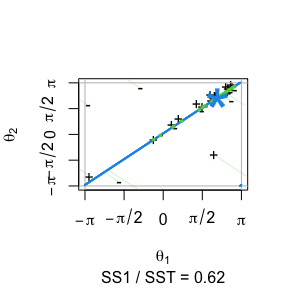# Variance explained
fit$var_exp #>  0.6191477 1.0000000 The produced scores allow the identification of outliers and “rectify” the original sample. plot(fit$scores, xlim = c(-pi, pi), ylim = c(-pi, pi), pch = 16, axes = FALSE,
xlab = "Scores 1", ylab = "Scores 2")
sdetorus::torusAxis()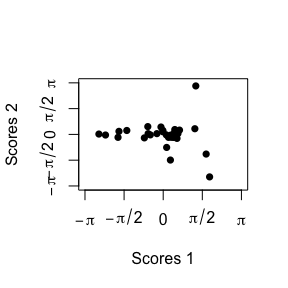## Japanese earthquakes

The following dataset represents pre-earthquake direction of steepest descent and the direction of lateral ground movement after an earthquake in Noshiro, Japan. We use TR-PCA to unveil the behavior and explain the variability of the earthquake phenomena.

data("earthquakes")
earthquakes <- sdetorus::toPiInt(earthquakes)
plot(earthquakes, xlim = c(-pi, pi), ylim = c(-pi, pi),
xlab = expression(theta), ylab = expression(theta), axes = FALSE)
sdetorus::torusAxis()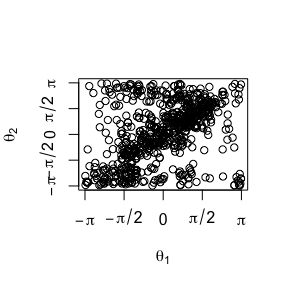The dataset is modeled with the best-fitting model among a Bivariate Wrapped Cauchy and a Bivariate Sine von Mises. Once the distribution is determined, the connected component of the density ridge can be computed. This flexible first principal component explains $$66\%$$ of the variance and shows a clear linear trend between both studied angles.

fit_earthquakes <- ridge_pca(x = earthquakes)
fit_earthquakes$var_exp #>  0.6554334 1.0000000 show_ridge_pca(fit_earthquakes)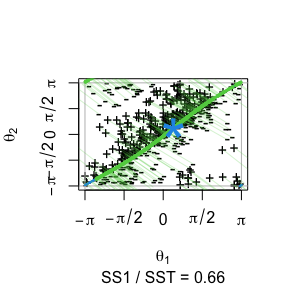plot(fit_earthquakes$scores, pch = 16, xlim = c(-pi, pi), axes = FALSE,
ylim = c(-pi, pi), xlab = "Scores 1", ylab = "Scores 2")
sdetorus::torusAxis()